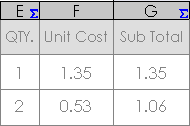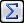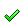Detailing OverviewSetting Detailing OptionsModel ItemsModel Items PropertyManagerAdd or Update a StyleTables OverviewGeneral TablesTable Cell, Row, or Column ToolbarAdding Symbol Text to BOM or Table CellsGeneral Format Precision in Table EquationsDisplaying the Sum of Column Cells in a TableTotaling Column Cells in a TableDefining Table Equations by Mathematical Operations on Custom PropertiesCombining Properties in Table EquationsTesting Conditions to Populate TablesTable Equation EditorTable AnchorsTable PropertyManagerGlossary

# Table Equation Editor

You can use the equation editor to include calculated quantities or simple logic in a table or BOM cell, or in a BOM column.

Equations in cells or columns are indicated with an equation symbol.To open the equation editor from a table or BOM cell: Select a cell. Click Equation(Table format toolbar). To open the equation editor from a BOM column: Double-click the top of a BOM column, just above the column heading. Select Equation from the Column type list, and click Equation.

## Using the Equation Editor

To use the equation editor:

1. Select a mathematical or logical operator from the Functions list.
2. Select table cells on which to operate and save the equation.
When creating equations for tables, you can:
• Type the argument for an operator between parentheses.
• Select a custom property on which to operate from the Custom Properties list.
• Click cell values (such as B3 or A1) to use in an equation. For example, B3*.08.
• For columns of BOM tables only, select the column on which to operate from the Columns list.
• Select a precision for numerical calculations from the Precision list.
Precision must precede the equation. For example, to set the precision to 2 decimal places for the product of cell A1 and .75, use {2}A1*.75.

## Example Equation

You can calculate the total of the items in a BOM column and display this value in another BOM table cell.

1. Select a blank table cell in the BOM and open the equation editor.
2. Type or select SUM from the Functions list.
3. Type the BOM column or cells to total. For example, to find the sum of the items in a column:
1. Click the first item in the column.
2. Type :.
3. Click the last item in the column.

The equation code appears in the equation text area.

4. Click.

Provide feedback on this topic

SOLIDWORKS welcomes your feedback concerning the presentation, accuracy, and thoroughness of the documentation. Use the form below to send your comments and suggestions about this topic directly to our documentation team. The documentation team cannot answer technical support questions. Click here for information about technical support.

* Required

 *Email: Subject: Feedback on Help Topics Page: Table Equation Editor *Comment: * I acknowledge I have read and I hereby accept the privacy policy under which my Personal Data will be used by Dassault Systèmes

Print Topic

Select the scope of content to print:

x

We have detected you are using a browser version older than Internet Explorer 7. For optimized display, we suggest upgrading your browser to Internet Explorer 7 or newer.

Never show this message again
x

Web Help Content Version: SOLIDWORKS 2013 SP05

To disable Web help from within SOLIDWORKS and use local help instead, click Help > Use SOLIDWORKS Web Help.

To report problems encountered with the Web help interface and search, contact your local support representative. To provide feedback on individual help topics, use the “Feedback on this topic” link on the individual topic page.